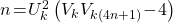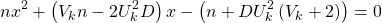Neşe Ömür and Zehra Betül Gür
Notes on Number Theory and Discrete Mathematics
Print ISSN 1310–5132, Online ISSN 2367–8275
Volume 26, 2020, Number 2, Pages 198—204
DOI: 10.7546/nntdm.2020.26.2.198-204

## Details

### Authors and affiliations

Neşe ÖmürDepartment of Mathematics, University of Kocaeli
41380 Izmit Kocaeli, Turkey

Zehra Betül GürDepartment of Mathematics, University of Kocaeli
41380 Izmit Kocaeli, Turkey

### Abstract

In this paper, we consider generalized Fibonacci quadratics and give solutions of them under certain conditions. For example, for odd numberunder condition, the equationhas rational roots.

### Keywords

• Generalized Fibonacci numbers
• Pythagorean triplet

• 11B39
• 11B50
• 97F40

### References

1. Horadam, A. F. (1965). Basic properties of a certain generalized sequence of numbers, The Fib. Quart., 3 (3), 161–176.
2. Kılıç, E., & Ömür, N. (2010). Conics characterizing the generalized Fibonacci and Lucas sequences with indices in arithmetic progressions, Ars Com., 94, 459–464.
3. Kılıç, E., & Stanica, P. (2009). Factorizations and representations of second linear recurrences with indices in arithmetic progressions, Bol. Mex. Math. Soc., 15 (3), 23–35.
4. Kılıç, E., & Stanica, P. (2011). Factorizations of binary polynomial recurrences by matrix methods, Rocky Mount. J. Math., 41 (4), 1247–1264.
5. Long, C., Cohen, G., & Langtry, T. (1993). Arithmetic sequences and second order
recurrences, Appl. of Fib. Num., 5, 449–458.
6. Mahanthappa, M. K. (1991). Arithmetic sequences and Fibonacci quadratics, The Fib. Quart., 29 (4), 343–346.
7. Umansky, H. (1973). A Fibonacci quadratic, The Fib. Quart., 11, 221–222.

## Cite this paper

Ömür, N., & Betül Gür, Z. (2020). On generalized Fibonacci quadratics. Notes on Number Theory and Discrete Mathematics, 26 (2), 198-204, doi: 10.7546/nntdm.2020.26.2.198-204.